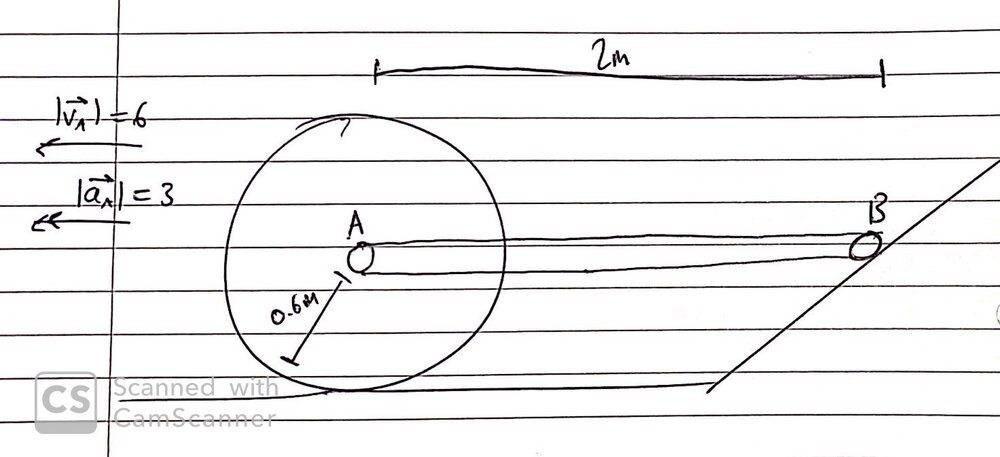# Dynamics homework -- Motion of a stick attached to a rolling disk

Serhatakguc0
Homework Statement:
Dynamic homework need help
Relevant Equations:
Velocity, Angular velocity, acceleration , angular acceleration
Hi everyone,

I need help for this homework. I'm a mechatronics engineering student and i want to solve this question but no matter how hard I try, I can't solve the question. Sorry for my bad english...

The disk connected to the AB stick with a length of 2 meters is rolled as shown in the figure without slipping. The velocity and acceleration of point A at the given moment moves to the left with VA = 6 m / s and aA = 3 m / s ^ 2, as shown in the figure, respectively.

A-)Find the velocity of point B and the angular velocity of point AB.

B-)Find the acceleration of point B and the angular acceleration of the AB bar.

C-)Find the angular velocity and angular acceleration of the disc.

Thanks.

Last edited:

Mentor
Welcome to the PF.What "relevant equations" do you think may apply? Can you show us a couple of your failed attempts to work on this problem? Can you show us your free body diagram (FBD)? Thanks.

Homework Helper
Gold Member
2022 Award
... and please post the diagram provided with the question. If you cannot do that, describe it thoroughly.

Mentor
... and please post the diagram provided with the question. If you cannot do that, describe it thoroughly.
That's weird. The OP originally did have a diagram included. It was confusing, but it was there.

@Serhatakguc0 -- Did you delete the figure for a reason?

Until @Serhatakguc0 gets back, I did manage to scribble something down of the diagram he had:•berkeman
Serhatakguc0
Hi again,
Welcome to the PF.What "relevant equations" do you think may apply? Can you show us a couple of your failed attempts to work on this problem? Can you show us your free body diagram (FBD)? Thanks.

That is my work on this problem and I'm so sorry about delete the diagram i want to delete it in attach files but i do a mistake.

#### Attachments

Homework Helper
Gold Member
2022 Award
Hi again,

That is my work on this problem and I'm so sorry about delete the diagram i want to delete it in attach files but i do a mistake.